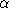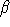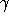## RootOf_2

### Definition

Concept to represent algebraic numbers of degree up to 2 over a RingNumberType denoted as RT.

### Creation

RootOf_2 make_root_of_2 ( RT a, RT b, RT c, bool s)
Returns the smaller or larger root of the equation aX2+bX+c=0, if s is true or false, respectively.
 Precondition: b2-4ac0
RootOf_2 make_root_of_2 ( FT a, FT b, FT c, bool s)
Returns the smaller or larger root of the equation aX2+bX+c=0, if s is true or false, respectively.
 Precondition: b2-4ac0
RootOf_2 make_root_of_2 ( FT alpha, FT beta, FT gamma)
Constructs the number+sqrt().
 Precondition:0

### Operations

The comparison operators ==, !=, <, >, <=, >= as well as the sign and compare functions need to be provided to compare elements of types RootOf_2, RT and FT.

In addition, the following operations must be provided:

 RootOf_2 RT a + RootOf_2 r RootOf_2 FT a + RootOf_2 r RootOf_2 RootOf_2 r + RT a RootOf_2 RootOf_2 r + FT a

as well as

 RootOf_2 RootOf_2 r + RootOf_2 r Addition of two algebraic numbers, in the special case when they have been constructed from equations having the same discriminant.

and similarly for operators -, * and /.

 RootOf_2 square ( RootOf_2 r) RootOf_2 inverse ( RootOf_2 r) RootOf_2 make_sqrt ( RT r) RootOf_2 make_sqrt ( FT f)

### Has Models

double (non exact)
CGAL::Root_of_2, etc